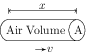# Work and Power

## Problems from IIT JEE

Problem (IIT JEE 2000): A wind-powered generator converts wind energy into electric energy. Assume that the generator converts a fixed fraction of the wind energy intercepted by its blades into electric energy. For wind speed $v$, the electric power output will be proportional to,

1. $v$
2. $v^2$
3. $v^3$
4. $v^4$

Solution:Let $\rho$ be the density of the air and $A$ be the cross-section area of the blades. Consider a cylindrical volume $V$ with cross-sectional area $A$ and length $x$ (see figure). The mass of air contained in volume $V$ is $m=\rho A x$ and its kinetic energy is, \begin{align} K=\tfrac{1}{2}mv^2=\tfrac{1}{2}\rho A x v^2.\nonumber \end{align} The time taken by this volume to intersect the blades is $\Delta t=x/v$. The air transfers its entire kinetic energy to the blades (assuming $100\; \%$ efficiency). Thus, the power generated is, \begin{align} P=\frac{K}{\Delta t}=\frac{1}{2}\rho A v^3.\nonumber \end{align}